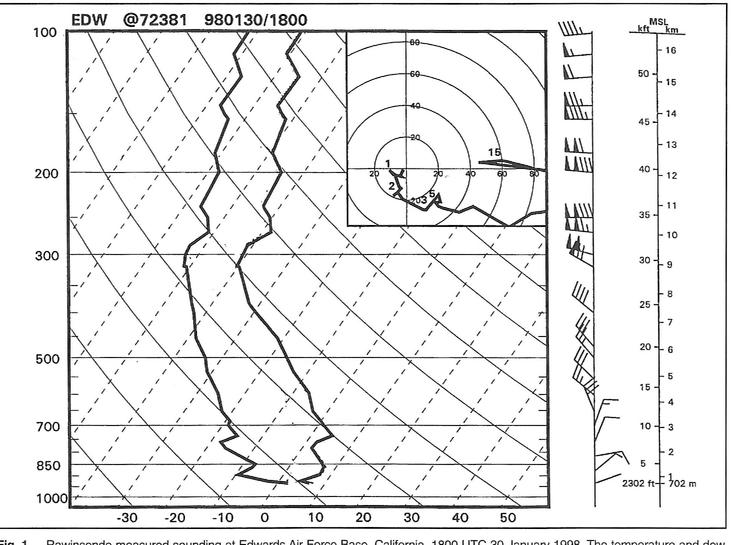# Unbalanced flow

## Text

The macro motion and state of the gas is the flow of the microscopic physicochemical process. In high temperature or exterior excitation sources (such as lasers), the micro freedom (flat, rotation, vibration, and electronic state) of gas molecules will be transmitted to each other due to a certain degree of excitation, and the molecules and atoms are transmitted. Chemical and ionization reactions. At this time, the macro motion and state of the gas will affect each other with the microscopic chemical process. Therefore, the characteristics of the gas medium associated with the molecular property are no longer maintained, such as the basic equation and relationship of the gas motion, such as continuous equations, energy equations, state equations, and ratios. Thermal relationship is also different from the basic equations and relationships in the usual gas dynamics, and must be corrected. Describe the microscopic chemical process, considering the impact of gas macro motion and state changes. The feature amount describing the degree of macro flow and microscopic process is the first class Damkeler number, defined as

as a feature flow time;
The representative time required to chemically react between the energy transfer or component of the molecule, that is, the characteristic relaxation time. When the number of DAs corresponding to a certain micro equation is close to 1, the microscopic process is in an unbalanced state, and this flow is also referred to as unbalanced flow.## Classification

1 flat and rotational non-balance stream: If

minimal or flow field physical quantity change gradient is extremely large In the hike and boundary layer, the positive and rotational non-equilibrium effects are generally tacky, thermal conductivity and diffusion. When it is in a non-equilibrium state, the speed distribution of gas molecules is no longer a Maxwell distribution. The study of positive non-balanced stream is mainly to solve the Bolzman equation in molecular motion theory, which is the theoretical basis for calculation of gas transport characteristics.

2 vibration and chemical non-balance stream: The characteristic relaxation time of vibration energy transfer and chemical reactions is far more than a long-equilibrium stream than the flat relaxation time. For a stream of stream fields and a non-balanced flow of vibration and chemical reactions (or ionization) in flow fields such as gas flow lasers, gas kinetic, vibration, and chemical reactive relaxation processes are simultaneously considered. The case where vibration energy transmission, and chemical constitutive changes are determined by the rate equation. Describe the rate equation that the vibration energy transfer is:

as the vibration relaxation time; EV and
are vibration energy and their balanced values, respectively. The type of vibration energy transfer and its relaxation time are determined, the gas chemical composition, chemical reaction and the relaxation time are determined, and the calculation of statistical mechanics, quantum mechanics, and chemical reaction kinetics is required, in particular, the experiment is required.

3 Radiation non-balance stream: The energy transfer caused by radiation is a non-balance process, and the movement of the gas is a non-insulating process. It is therefore necessary to consider the interaction between gas motion, radiation transmission, and unbalanced relaxation process (e.g., ionization, dissociation, and chemical reactions).

If the number of Dα of a micro process is much greater than or less than 1, the microscopic process is in a balanced or freezing state during the flow, and the corresponding flow is referred to as a balance flow and freezing flow. In balance flow (DA "1), the energy transfer between molecular microfearing is extremely fast, and the chemical reaction is extremely fast, almost every instant gas is in a thermodynamic equilibrium state that is adapted to the macro state, and in the flow field Any minor group is satisfied with the same thermodynamic relationship with a complete thermodynamic balance. Therefore, the balance thermodynamic relationship can be combined into the equation of the gas dynamics, and the necessary additional relationship between the contact state variable can be provided. For freezing flow (DA "1), the energy transfer between molecular microscopic freedom is extremely slow, the chemical reaction rate is extremely slow, and the energy transfer between microfearing in the flow field, the chemical reaction presents" frozen "state, that is, gas Microscopic freedom energy and gas components are fixed, equal to their respective initial values. Thus, the gas mixture has thermal and thermal properties. The thermal complete gas refers to a gas that satisfies the state equation PV = NRT, and the formulas P, T, R are the pressure, thermodynamic temperature, and general gas constants of the gas; N is the molecular number of molecules of the volume V. The heat complete gas is a gas (see a complete gas). In this way, the gas can be obtained as a constant value suitable for the corresponding situation, freezing, etc., which can be obtained by parameters such as heat (regular pressure ratio heat

, set to heat
, and more thermogravime). The analysis of the flow field can be done according to the extent of the full gas.

Research on balancing flow, unbalanced flow and freezing flow, has theoretical and actual research on high ultrasound speed winding flow field, strong waves, high temperature plasma, pneumatic laser and chemical laser, etc. significance.

Related Articles